# Fraction calculator

The calculator performs basic and advanced operations with fractions, expressions with fractions combined with integers, decimals, and mixed numbers. It also shows detailed step-by-step information about the fraction calculation procedure. Solve problems with two, three, or more fractions and numbers in one expression.

## Result:

### 3/4 * 1/3 = 1/4 = 0.25

Spelled result in words is one quarter.

### How do you solve fractions step by step?

1. Multiple: 3/4 * 1/3 = 3 · 1/4 · 3 = 3/12 = 1 · 3/4 · 3 = 1/4
Multiply both numerators and denominators. Result fraction keep to lowest possible denominator GCD(3, 12) = 3. In the following intermediate step, cancel by a common factor of 3 gives 1/4.
In other words - three quarters multiplied by one third = one quarter.

#### Rules for expressions with fractions:

Fractions - use the slash “/” between the numerator and denominator, i.e., for five-hundredths, enter 5/100. If you are using mixed numbers, be sure to leave a single space between the whole and fraction part.
The slash separates the numerator (number above a fraction line) and denominator (number below).

Mixed numerals (mixed fractions or mixed numbers) write as non-zero integer separated by one space and fraction i.e., 1 2/3 (having the same sign). An example of a negative mixed fraction: -5 1/2.
Because slash is both signs for fraction line and division, we recommended use colon (:) as the operator of division fractions i.e., 1/2 : 3.

Decimals (decimal numbers) enter with a decimal point . and they are automatically converted to fractions - i.e. 1.45.

The colon : and slash / is the symbol of division. Can be used to divide mixed numbers 1 2/3 : 4 3/8 or can be used for write complex fractions i.e. 1/2 : 1/3.
An asterisk * or × is the symbol for multiplication.
Plus + is addition, minus sign - is subtraction and ()[] is mathematical parentheses.
The exponentiation/power symbol is ^ - for example: (7/8-4/5)^2 = (7/8-4/5)2

#### Examples:

subtracting fractions: 2/3 - 1/2
multiplying fractions: 7/8 * 3/9
dividing Fractions: 1/2 : 3/4
exponentiation of fraction: 3/5^3
fractional exponents: 16 ^ 1/2
adding fractions and mixed numbers: 8/5 + 6 2/7
dividing integer and fraction: 5 ÷ 1/2
complex fractions: 5/8 : 2 2/3
decimal to fraction: 0.625
Fraction to Decimal: 1/4
Fraction to Percent: 1/8 %
comparing fractions: 1/4 2/3
multiplying a fraction by a whole number: 6 * 3/4
square root of a fraction: sqrt(1/16)
reducing or simplifying the fraction (simplification) - dividing the numerator and denominator of a fraction by the same non-zero number - equivalent fraction: 4/22
expression with brackets: 1/3 * (1/2 - 3 3/8)
compound fraction: 3/4 of 5/7
fractions multiple: 2/3 of 3/5
divide to find the quotient: 3/5 ÷ 2/3

The calculator follows well-known rules for order of operations. The most common mnemonics for remembering this order of operations are:
PEMDAS - Parentheses, Exponents, Multiplication, Division, Addition, Subtraction.
BEDMAS - Brackets, Exponents, Division, Multiplication, Addition, Subtraction
BODMAS - Brackets, Of or Order, Division, Multiplication, Addition, Subtraction.
GEMDAS - Grouping Symbols - brackets (){}, Exponents, Multiplication, Division, Addition, Subtraction.
Be careful, always do multiplication and division before addition and subtraction. Some operators (+ and -) and (* and /) has the same priority and then must evaluate from left to right.

## Fractions in word problems:

• ExpressionsLet k represent an unknown number, express the following expressions: 1. The sum of the number n and two 2. The quotient of the numbers n and nine 3. Twice the number n 4. The difference between nine and the number n 5. Nine less than the number n
• Ms. SheppardMs. Sheppard cuts ½ of a piece of construction paper. She uses ⅙ of the piece to make a flower. What fraction of the sheet of paper does she use to make the flower?
• Zangmo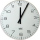Zangmo can read a chapter of a novel in 1/4 h. How long will it take her to read 8 chapters?
• Yellow tangs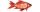In an aquarium 2/5 of the fish are surgeonfish.  Of these 3/4 are yellow tangs.  What fraction of all fish in the aquarium are yellow tangs?
• Jeff rode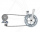Jeff rode his bike around a bike trail that was 1/3 of a mile long. He rode around the trail 9 times. Write a fraction greater than 1 for the distance. How many miles did Jeff ride?
• Visit to grandfatherShane's family traveled 3/10 of the distance to his grandfather’s house on Saturday. They traveled 4/7 of the remaining distance on Sunday. What fraction of the total distance to his grandfather’s house was traveled on Sunday?
• Lunch timeIn a cafeteria, 3/10 of the students are eating salads, and 3/5 are eating sandwiches. There are 30 students in the cafeteria. How many students are eating lunches other than salads or sandwiches?
• CerealsAri and Joey share a 30-ounce box of cereal. By the end of the week, Ari has eaten 3/10 of the box, and Joey has eaten 3/5 of the box of cereal. How many ounces are left in the box?
• Sally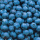Sally is making 15 individual blueberry cheesecakes. How many pounds of blueberries will be in each cheesecake if 5 1/4 pounds of blueberries are divided equally among them?
• Length 7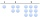Length equals 7/6 inch in width equals 7/9 inches the area is?
• NumbersDetermine the number of all positive integers less than 4183444 if each is divisible by 29, 7, 17. What is its sum?
• Ratio - proportion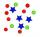Reduce the number 16 in proportion 3:2 5:4 11:8To three-eighths of one third, we add five quarters of one half and multiply the sum by four. How much will we get?